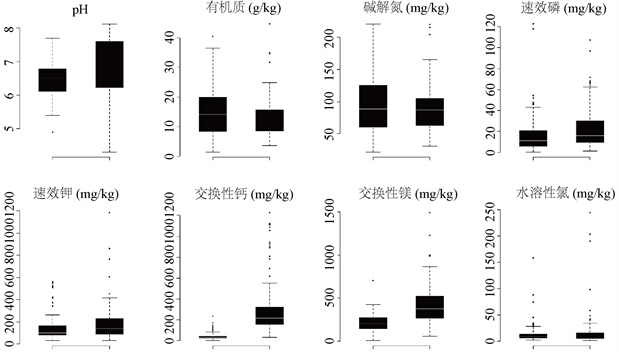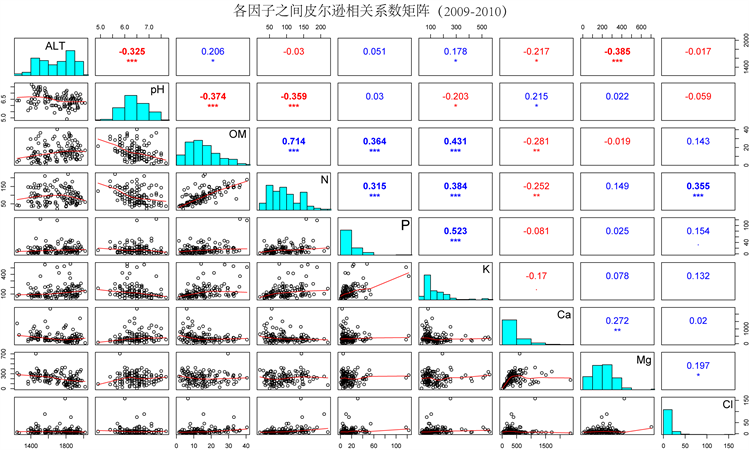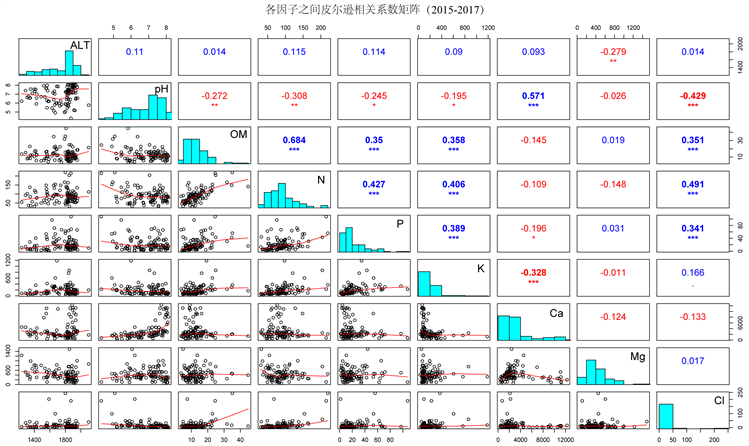﻿ 仁和烟区土壤肥力状况时空变化综合评价

# 仁和烟区土壤肥力状况时空变化综合评价Comprehensive Evaluation of Soil Fertility and Spatial-Temporal Characteristics in Sichuan’s Renhe Tobacco-Growing Areas

Abstract: Harmonious soil nutrient and highly comprehensive fertility are guaranteed of high quality and high yield of crops. In this paper, principal component analysis and equidistant cluster analysis were used to evaluate the status of soil nutrients and fertility. The results showed that: 1) The pH value and available nitrogen were suitable; organic matter and water-soluble chlorine were low; available phosphorus, available potassium, exchangeable calcium and exchangeable magnesium were rich. From 2011 to 2017, pH value was slightly increased, organic matter and water-soluble chlorine ware decreased gradually; available phosphorus, available potassium, exchangeable calcium and exchangeable magnesium increased significantly. 2) The IFI value of the whole soil samples range from 0.19 to 0.82 in 2017 year, with the average IFI value was 0.52, which was higher than that in 2010, but the coefficient of variation was lower than that in 2010. Comparing with 2010 year, IFI value of medium and low altitude in 2017 year was increased, while that of high altitude was decreased. The soil integrated fertility index of different altitudes was medium altitude > high altitude > low altitude. 3) Our results also showed that the contribution of various indexes of soil nutrients to the IFI value varied greatly, which indicated that the application of fertilizers in successive years led to the aggravation of soil nutrient imbalance.

1. 引言

2. 材料与方法

2.1. 样品采集与分析

2.2. 数据分析与评价方法

$IFI=\underset{1}{\overset{n}{\sum }}Wi×Ni$ (1)

3. 结果与分析

3.1. 土壤养分状况描述性统计分析Figure 1. Descriptive statistical of soil fertility indexes in Renhe tobacco-growing areas

2009~2010年结果显示，总体土壤有机质平均含量为15.23 ± 8.88 g∙kg−1，且与海拔呈现明显正相关，而2017年显示总体土壤有机质平均含量为12.09 ± 4.88 g∙kg−1，且与海拔无相关关系。可见两次普查之间施肥措施致使土壤有机质降低。两次普查显示土壤碱解氮、水溶性氯保持稳定，基本无变化，且其含量与海拔无明显关系。2017年土壤速效磷、速效钾、交换性钙、交换性镁四个指标明显高于2010年，总体均值分别提高50.0%、36.4%、695.0%和95.2%；2010年土壤速效钾含量随海拔升高而升高，交换性镁、交换性钙则随海拔升高而降低，2017年则土壤速效磷、速效钾在1600~1800 m海拔范围最高，交换性钙在此海拔最低，交换性镁则随海拔升高而降低。

3.2. 土壤养分状况时空变化及综合分析

3.2.1. 隶属度函数确定及赋值

$f\left(x\right)=\left\{\begin{array}{l}1.0\text{ }\text{ }\text{ }\text{ }\text{ }\text{ }\text{ }\text{ }\text{ }\text{ }\text{ }\text{ }\text{ }\left(x\ge {x}_{2}\right)\\ 0.9×\left(x-{x}_{1}\right)/\left({x}_{2}-{x}_{1}\right)+0.1\text{ }\text{ }\text{\hspace{0.17em}}\text{\hspace{0.17em}}\left({x}_{1}\le x<{x}_{2}\right)\\ 0.1\text{ }\text{ }\text{ }\text{ }\text{ }\text{ }\text{ }\text{ }\text{ }\text{ }\text{ }\text{ }\text{ }\left(x\ge {x}_{2}\right)\end{array}$ (2)

$f\left(x\right)=\left\{\begin{array}{l}0.1\text{ }\text{ }\text{ }\text{ }\text{ }\text{ }\text{ }\text{ }\text{ }\text{ }\text{ }\text{ }\text{ }\left(x<{x}_{1},x>{x}_{4}\right)\\ 0.9×\left(x-{x}_{1}\right)/\left({x}_{2}-{x}_{1}\right)+0.1\text{ }\text{ }\text{\hspace{0.17em}}\text{\hspace{0.17em}}\left({x}_{1}\le x<{x}_{2}\right)\\ 1.0\text{ }\text{ }\text{ }\text{ }\text{ }\text{ }\text{ }\text{ }\text{ }\text{ }\text{ }\text{ }\text{ }\left({x}_{2}\le x\le {x}_{3}\right)\\ 1.0-0.9×\left(x-{x}_{4}\right)/\left({x}_{2}-{x}_{4}\right)\text{ }\text{ }\left({x}_{3} (3)

3.2.2. 肥力指标权重值Table 1. Types of membership function and threshold values of each soil fertility indexTable 2. Communalities and weight value of each fertility index

3.2.3. 土壤肥力综合评价

4. 讨论与结论

4.1. 各个肥力指标时空变化Table 3. Comprehensive evaluations of soil fertility status

2010年至2017年交换性钙、交换性镁含量大幅度上升，尤其是交换性钙，总体上升幅度达到695%，>1800 m海拔高度交换性钙增幅更是达到962%，不论是增幅还是含量，本研究结果与前人研究结果基本一致  这与土壤pH值结果完全一致，基本可以肯定是受到酸化土壤改良措施的影响，该烟区主要的改良土壤酸化措施为使用石灰，该改良剂使用广泛、改良效果好，同时可补充土壤钙含量，但过量和不合理使用石灰会引起短期内土壤pH值急剧升高 ，并由此导致部分土壤碱化，因此在施用石灰时应该注意用量和频率。

4.2. 海拔与各个指标的相关关系

2009~2010年结果表明，土壤有机质、速效钾含量与海拔显著正相关，而交换性钙、交换性镁、pH与海拔显著负；2015~2017年结果显示，交换性镁与海拔显著负相关，其他因子均不相关(图2)。海拔是影响山地植烟土壤的重要因子，土壤理化性质随海拔高度而变化，如低海拔土壤碱解氮、有机质、有效硫含量明显低于中高海拔 。

pH值与交换性钙显著正相关，与有机质、碱解氮极显著负相关。植烟土壤pH值与土壤养分的关系已经有大量报道  ，且已有报道结果并不完全一致，这说明样本量大小、土壤母质、气候条件以及种植制度等对土壤pH值与养分之间关系均有影响，但即便如此，所有报道均表明土壤pH值与土壤交换性钙、交换性镁显著正相关，这与本研究结果一致，说明在更大尺度范围内，交换性钙镁与土壤pH值的关系会更加紧密。

4.3. 综合肥力评价指标值时空变化Figure 2. Correlation coefficient among elevation, pH and soil nutrientsFigure 3. IFI values of different elevation in both years

NOTES

*通讯作者。

 Sehnitzer, M. (1991) Soil Organic Matter—The Next 75 Years. Soil Science, 151, 41-58.
https://doi.org/10.1097/00010694-199101000-00008

 曹志洪, 周建民. 中国土壤质量[M]. 北京: 科学出版社, 2008: 30-39.

 马强, 宇万太, 赵少华, 等. 黑土农田土壤肥力质量综合评价[J]. 应用生态学报, 2004, 15(10): 1916-1920.

 王林, 卢秀萍, 肖汉乾, 等. 浏阳植烟土壤肥力状况的综合评价[J]. 河南农业大学学报, 2006, 40(6): 597-600.

 许自成, 刘国顺, 刘金海, 等. 铜山烟区生态因素和烟叶质量特点[J]. 生态学报, 2005, 25(7): 1748-1753.

 王晖, 邢小军, 许自成. 攀西烟区紫色土pH值与土壤养分的相关分析[J]. 中国土壤与肥料, 2007(6): 19-22.

 鲁如坤. 土壤农业化学分析方法[M]. 北京: 中国农业科学技术版社, 2000.

 鲍士旦. 土壤农化分析[M]. 第3版. 北京: 中国农业出版社, 2000.

 周学文, 赵小敏, 胡国瑞, 等. 南方丘陵地区水田土壤养分变异分析[J]. 江西农业大学学报, 2009, 31(5): 919-926.

 赵辉, 邵素华, 谢东坡. 分析数据中离群值的处理方法[J]. 周口师范学院学报, 2004, 21(5): 70-71, 115.

 刘琼峰, 李明德, 吴海勇, 等. 张家界烟区不同海拔高度植烟土壤特征与综合评价[J]. 中国农学通报, 2013, 29(11): 132-138.

 郭明全, 胡建新, 张宗锦. 攀枝花烟区土壤pH分布特点及其与土壤有效养分的关系[J]. 西南农业学报, 2012, 25(4): 1338-1341.

 曾庆宾, 杨军伟, 徐祥玉, 等. 攀枝花烟区土壤酸碱及钙、镁变化分析[J]. 湖北农业科学, 2017, 56(6): 1052-1055.

 杨军伟, 曾庆宾, 张瑞平, 等. 攀枝花市烟区土壤有机质及氮素变化分析[J]. 湖北农业科学, 2016, 55(9): 2195-2197.

 田飞, 罗建新, 严红星, 等. 常德市植烟土壤养分状况与评价[J]. 粮食科技与经济, 2019, 44(1): 133-136.

 孟赐福, 傅庆林, 水建国, 等. 浙江中部红壤施用石灰对土壤交换性钙、镁及土壤酸度的影响[J]. 植物营养与肥料学报, 1999, 5(2): 129-136.

 陈江华, 刘建利, 李志宏. 中国植烟土壤及烟草养分综合管理[M]. 北京: 科学出版社, 2008.

 刘刚, 李浩, 董贤春, 等. 宜昌植烟土壤养分含量变化特征[J]. 安徽农业科学, 2019, 47(16): 187-190, 268.

 胡国松, 郑伟, 王震东. 烤烟营养原理[M]. 北京: 科学出版社, 2000: 55-57.

 李强, 周冀衡, 何伟, 等. 中国主要烟区烤烟氯含量区域特征研究[J]. 中国土壤与肥料, 2010(2): 49-54.

 张翔, 范艺宽, 黄元炯, 等. 烤烟吸收氯的主要来源及其在体内分布的研究[J]. 土壤肥料, 2006(2): 62-64.

 闫芳芳, 苏兆俊, 张映杰, 等. 攀枝花烟区土壤水溶性氯含量及其氯肥适宜用量研究[J]. 天津农业科学, 2018, 24(11): 69-74.

Top